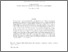# Stochastic volatility implies fourth-degree risk dominance: Applications to asset pricing

Gollier, Christian (2018) Stochastic volatility implies fourth-degree risk dominance: Applications to asset pricing. Journal of Economic Dynamics and Control, 95. pp. 155-171.Preview
Text
Official URL: http://tse-fr.eu/pub/31266

## Abstract

We demonstrate that increasing the risk surrounding the variance of future consumption generates a fourth-degree risk deterioration in future consumption, yielding an increase in its excess kurtosis. Its impact on the equilibrium risk premium is thus positive if only if the fourth derivative of the utility function is negative. Its impact on interest rates is negative only if its fifth derivative is positive. We also show that the persistence of shocks to the variance of the consumption growth rate, as assumed in long-run risk models, has no effect on the term structure of the variance ratio which remains flat in expectation, but it makes the term structure of the annualized fourth cumulant of log consumption increasing. It generates term structures of interest rates and risk premia that are respectively decreasing and increasing under constant relative risk aversion. Using recursive preferences does not qualitatively modify these results, which are counterfactual. However, the persistence of shocks to the variance of changes in log consumption is supported by the observation that their annualized 4th cumulant exhibits an increasing term structure over the period 1947Q1-2016Q4 in the United States.

Item Type: Article English 2018 Yes Long-run risk, fourth-degree stochastic dominance, temperance, recursive utility, kurtosis B- ECONOMIE ET FINANCE TSE-R (Toulouse) UT1 11 Sep 2018 14:20 24 Jul 2019 11:41 oai:tse-fr.eu:31266 http://publications.ut-capitole.fr/id/eprint/26239View Item